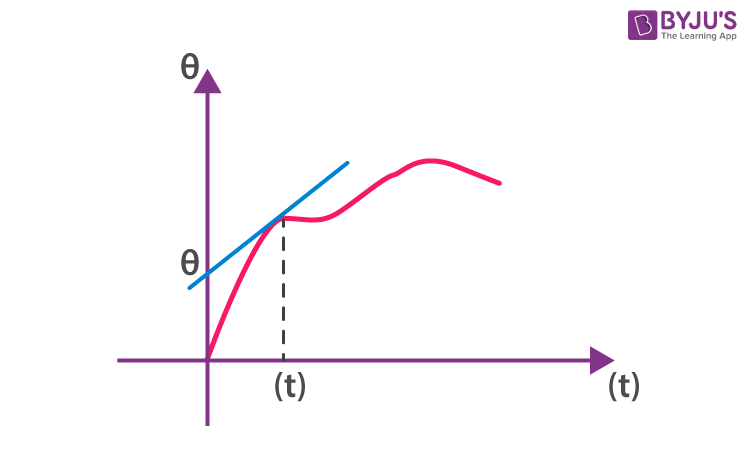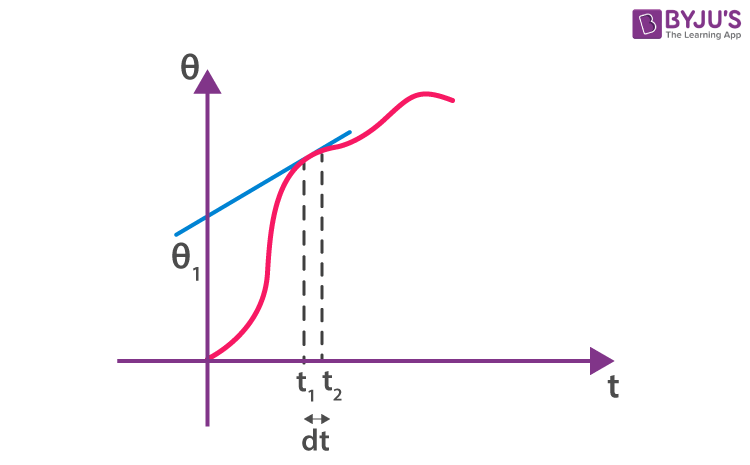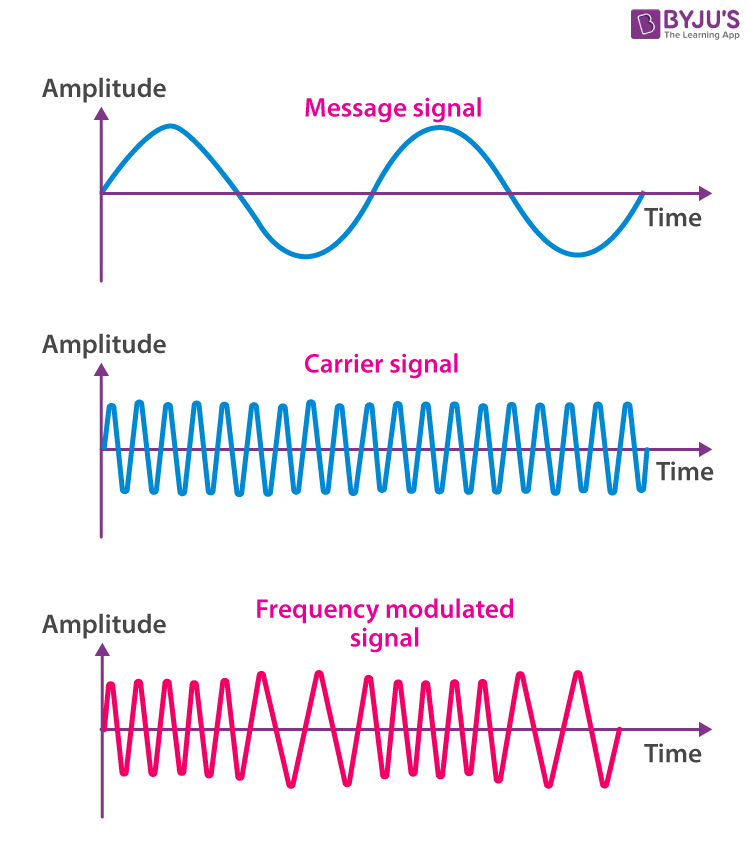Checkout JEE MAINS 2022 Question Paper Analysis : Checkout JEE MAINS 2022 Question Paper Analysis :

# Frequency Modulation

Frequency modulation or commonly referred to as FM is a common term that we hear in our daily lives. Today, Frequency modulation is used widely in radio communication and broadcasting. But have we wondered what it actually is or what is the technology and mechanism behind FM? We will try to understand what frequency modulation is in this lesson and also learn its mechanism as well as its applications.

## What is Frequency Modulation?

Frequency modulation is a technique or a process of encoding information on a particular signal (analogue or digital) by varying the carrier wave frequency in accordance with the frequency of the modulating signal. As we know, a modulating signal is nothing but information or message that has to be transmitted after being converted into an electronic signal.

Much like amplitude modulation, frequency modulation also has a similar approach where a carrier signal is modulated by the input signal. However, in the case of FM, the amplitude of the modulated signal is kept or it remains constant.

The frequency modulation index is mostly over 1 and it usually requires a high bandwidth at a range of 200 kHz. FM operates in a very high-frequency range normally between  88 to 108 Megahertz. There are complex circuits with an infinite number of sidebands that help in receiving high-quality signals with high sound quality.

Meanwhile, broadcast stations in the VHF portion of the frequency spectrum between 88.5 and 108 MHz often use large values of deviation (±75 kHz). This is known as wide-band FM (WBFM). Even though these signals support high-quality transmissions they do occupy a large amount of bandwidth. Normally, 200 kHz is allowed for each wide-band FM transmission. On the other hand, communications use very little bandwidth. Alternatively, narrowband FM (NBFM) often uses deviation figures of around ±3 kHz. Besides, narrow-band FM is mostly used for two-way radio communication applications.

## Applications of Frequency Modulation

If we talk about the applications of frequency modulation, it is mostly used in radio broadcasting. It offers a great advantage in radio transmission as it has a larger signal-to-noise ratio. Meaning, it results in low radio frequency interference. This is the main reason that many radio stations use FM to broadcast music over the radio.

Additionally, some of its uses are also found in radar, telemetry, seismic prospecting and in EEG, different radio systems, music synthesis as well as in video-transmission instruments. In radio transmission, frequency modulation has a good advantage over other modulation. It has a larger signal-to-noise ratio meaning it will reject radio frequency interferences much better than an equal power amplitude modulation (AM) signal. For this major reason, most music is broadcasted over FM radio.

## FM Modulators

There are several methods that can be used to generate either direct or indirect frequency-modulated signals.

• A voltage-controlled oscillator or Varactor diode oscillator:  A voltage-controlled oscillator can be used to form Direct FM modulation by directly feeding the message into the input of the oscillator. In the case of the varactor diode, we place this device within the tuned circuit of an oscillator circuit.
• Crystal oscillator circuit: Varactor diode can also be used within a crystal oscillator circuit wherein the signal needs to be multiplied in frequency, and only narrowband FM is attained.
• Phase-locked loop: This is an excellent method to generate frequency modulation signals. However, the constraints within the loop should be checked carefully and once everything is stable it offers an excellent solution.

## Frequency Modulation Equations

Frequency modulation equations mainly consist of a sinusoidal expression with the integral of the baseband signal that can be either a sine or cosine function.

It can be represented mathematically as;

m(t) = Am cos (ωmt + Ɵ) ……………… 1

m(t) → modulating signal

Where,

Am → Amplitude of the modulating signal.

ωm → Angular frequency of the modulating signal.

Ɵ → is the phase of the modulating signal.

Such as amplitude modulation, when we try to modulate an input signal (information), we need a carrier wave, we will experience

C(t) = Ac cos (ωct + Ɵ) ………….. 2

Angular modulation, which means ωc (or) Ɵ of the carrier wave starts varying linearly with respect to the modulating signal like amplitude modulation.

Case I: Any Instant

Modulating signal at any instant of time.C(t) = Ac cos (ωct + Ɵ)

For any particular instant (ωct + Ɵ) is not varying with respect to time the Ɵ becomes so it Ɵ0 = constant, then

ωc = also constant

If we draw a tangent for the given signal at any instant of time, the slope of the tangent gives ωc and the tangent when it cuts the Ɵ-axis gives Ɵ0 value.

Case II: For a Small Interval of Time

Now let us consider a small interval of time Δt = t2 – t1

Time interval, t1 < t < t2, we will look this into two particular instant of t1 and t2Let us consider an instant at (t1), if we draw a tangent to a given signal at (t1), the slope of curve instantaneous frequency (wi) at that particular instant

Similarly, intercept of the tangent with Ɵ – axis gives the instantaneous phase (Ɵi).  Likewise, we can get wi for any instant of the given curve. From this one thing is clear.

$$\begin{array}{l}{{\omega }_{i}}=\frac{d\theta }{dt}\end{array}$$

Ɵ – is the phase at the instant

We write this as a function of time (t), instantaneous frequency is

$$\begin{array}{l}\omega (t)=\frac{d\theta (t)}{dt}…………..3\end{array}$$
$$\begin{array}{l}\int\limits_{-\infty }^{t}{\omega (t)dt=\theta \,……………….\,4}\end{array}$$

Equation 3 and 4 gives the fundamental understanding of phase and frequency. If we try to modulate this signal, let us see what’s happening at any instant of time the signal phase is

$$\begin{array}{l}\theta (t)={{\omega }_{c}}t\int\limits_{-\infty }^{t}{k\,m(t)…………..(5)}\end{array}$$
[this is known as phase modulation]

Where,

k – constant

ωc → frequency of the carrier wave

m(t) → modulating signal

The insert signal in this phase becomes,

A cos Ɵ (t) = Ac cos [ωct + k m(t)]

If we need the frequency of the wave,

$$\begin{array}{l}\omega (t)=\frac{d\theta (t)}{dt}=\end{array}$$
ωc + km(t) ……….6 [this is known frequency modulation]

Where,

$$\begin{array}{l}\overset{\bullet }{\mathop{m}}\,(t)=\frac{d}{dt}m(t)\end{array}$$

As we know, the idea of frequency modulation, the frequency of the carrier wave must vary linearly with respect to particular signal as we can see it equation no 5. From this we get,

ω(t) = ωc + km(t)

If we do phase modulation it is nothing but a frequency modulation. When we do frequency modulation, we are differentiating the particular modulating signal which then automatically depicts it as phase modulation.

### Expression for Frequency Modulated Wave

As we know from amplitude modulation, we need two sine (or) cosine waves for modulation.

m(t) = Am cos (ωmt) and

c(t) = Ac cos (ωct)

or

m(t) = Am cos (2π fmt)

c(t) = Ac cos (2πfct)

Then frequency modulated wave will be;

fm(t) = fc + k Am cos (2π fm t )

fm (t) = fc + k m(t)

Where,

fm(t) = is frequency modulated wave

fc → frequency of the carrier wave

m(t) → modulating signal

k → proportionality constant.

## Frequencies in Frequency Modulation

In FM, variation (or) deviation in frequency the maximum deviation Δfmax

Δfmax = │fm(t)fc│

=│KAm cos(2π fmt)

The maximum deviation in frequency is K Am

Generally, frequency deviation is defined as the measure of the change in a carrier frequency produced by the amplitude of the input modulating signal.

## Modulation Index (μ)

Is the ratio of maximum deviation in frequency of the modulating signal.

$$\begin{array}{l}\mu =\frac{\Delta {{f}_{\max }}}{{{f}_{m}}}=\frac{{{K}_{{{A}_{m}}}}}{{{f}_{m}}}\end{array}$$

Also Read: Differences Between AM and FM

## Graphical Representation of Frequency Modulated WaveIf we observe the graph, we will notice that the frequency of a carrier increases when the amplitude of the input signal is increased. Here, the carrier frequency is maximum when the input signal is at its highest.  Meanwhile, the frequency of a carrier decreases if the amplitude of the modulating signal goes down.  What it means is that the carrier frequency is minimum when the input signal is at its lowest.

## Frequency Demodulation

When there is modulation, usually we need to successfully demodulate it and at the same time recover the original signal. In such cases, FM demodulator also known as FM discriminator or FM detector is used. While there are several types of FM demodulators, the main functionality of these devices is to convert the frequency variations of the input signal into amplitude variations of the output signal. The demodulators are used along with an audio amplifier, or possibly a digital interface.

 Advantages Disadvantages Less interference and noise. Equipment cost is higher. Has a large bandwidth. Power Consumption is less as compared to AM. More complicated receiver and transmitter Adjacent FM channels are separated by guard bands. The antennas for FM systems should be kept close for better communication.

## Amplitude Modulation vs Frequency Modulation

While FM and AM function in a similar manner the main difference lies in how their carrier waves are modulated. In the case of AM, the signal strength varies in order to incorporate sound information whereas for FM, the frequency at which the current changes direction per second for the carrier signal is varied. We will look at some of the differences between FM and AM below.

 Amplitude Modulation (AM) Frequency Modulation (FM) Frequency and phase remain the same Amplitude and phase remain the same Can be transmitted over a long distance but has poor sound quality. Better sound quality with higher bandwidth. The frequency range varies between 535 to 1705 kHz For FM it is from88 to 108 MHz mainly in the higher spectrum Signal distortion can occur in AM Less instances of signal distortion Consists of two sidebands An infinite number of sidebands Circuit design is simple and less expensive Circuit design is intricate and more expensive Easily susceptible to noise Less susceptible to noise

## Frequently Asked Questions on Frequency Modulation

### How does frequency modulation work?

The encoding of information in a carrier wave by modifying the wave’s instantaneous frequency is known as frequency modulation (FM). In the fields of computing, telecommunications, and signal processing, FM technology is frequently used.

### What is the advantage of frequency modulation over amplitude modulation?

FM was first introduced to reduce noise and increase radio reception quality because the frequency of a radio wave is less susceptible to noise than the amplitude. FM radio broadcasts have several times the bandwidth of AM signals to achieve this.

### How is the process of frequency modulation done?

The frequency of the radio carrier is modified in accordance with the amplitude of the incoming audio stream to form a frequency modulated signal.

### What are the different types of analog modulation systems?

Frequency Modulation, Amplitude Modulation, Phase Modulation are the different types of continuous wave analog modulation systems whereas PAM, PWM and PPM are the pulse analog modulation systems.

### What is frequency modulation?

Frequency modulation, is the method of changing the carrier signal’s instantaneous frequency in accordance with the message signal’s instantaneous amplitude.

### List the uses of modulation.

Modulation has the following uses.
Reduces antenna height.
Avoids signal mixing.
Increases communication range.
Multiplexing is possible.
Improves reception quality.

### What is frequency deviation?

The FM signal’s instantaneous frequency changes with time around the carrier frequency ωc. This indicates that the FM signal’s immediate frequency varies depending on the modulating signal. Frequency deviation is the maximum change in instantaneous frequency from the average frequency ωc.

### What are the drawbacks of frequency modulation over amplitude modulation?

In FM broadcasting, a significantly broader channel is required, approximately 200 kHz, compared to only 10 kHz in AM broadcasting. This is a significant restriction of FM. FM transmitting and receiving equipment, especially that utilised for modulation and demodulation, is more complicated and thus more expensive.

Test your Knowledge on Frequency modulation